Copyright © Michael Richmond. This work is licensed under a Creative Commons License.

# Magnetic Torques and Amp's Law

• A magnetic field exerts a force on a straight wire carrying current; it exerts a torque on a loop of wire carrying current.
• Torque causes an object to spin around a fixed axis.
• Each loop of current has a direction associated with it: its normal vector is perpendicular to the loop, in the direction given by the right thumb when the right fingers curl in the direction of the current.
• A magnetic field exerts a torque which tries to align the normal vector of a loop of current with the magnetic field.
• The size of the torque on a loop of current is
```       torque = (# turns) * (current) * (loop area) * (mag field) * sin(theta)
```
where theta is the angle between the magnetic field and the loop's normal vector.
• A loop carrying direct current will not keep spinning in a constant magnetic field; it will instead just wobble back and forth. DC motors must use a split-ring commutator to permit them to spin fully around.
• It is possible to create a magnetic field by running a current through a wire -- showing the close relationship between electricity and magnetism.
• Ampere's Law permits one to calculate the strength of a magnetic field created by current in several simple situations.
• The strength of a magnetic field around a long, straight wire is
```                         mu * current
mag field =  -------------------
2 * pi * distance
```
where mu is the magnetic permeability of free space:
```              mu     =  4 * pi * 10^(-7)  T*m/A

=  1.257 x 10^(-6)   T*m/A
```
• The strength of a magnetic field at the center of a loop of wire is
```                         N * mu * current
mag field =  -----------------
```
where N is the number of turns of wire in the loop.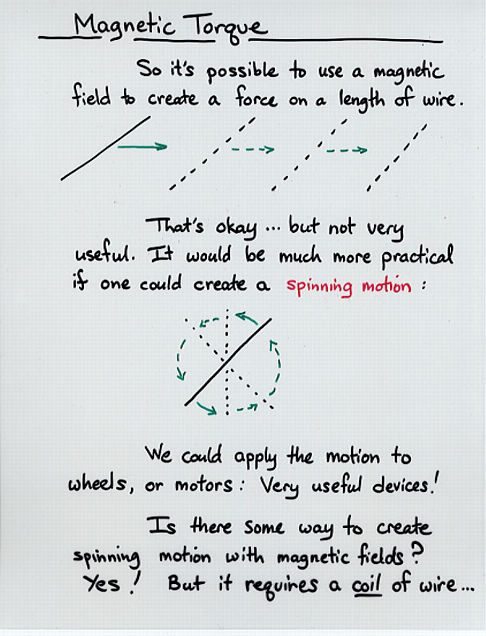Viewgraph 1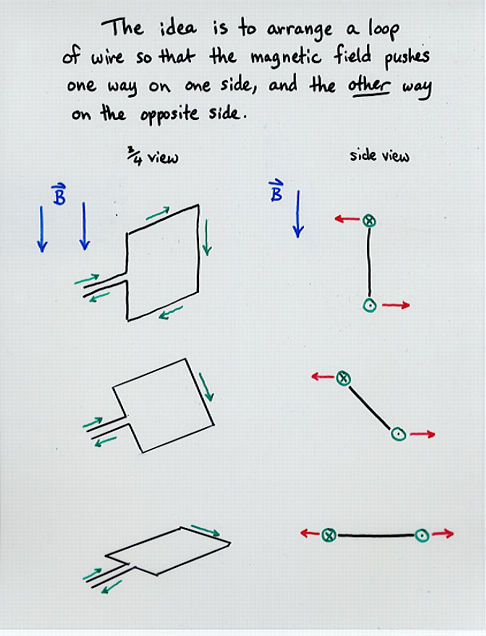Viewgraph 2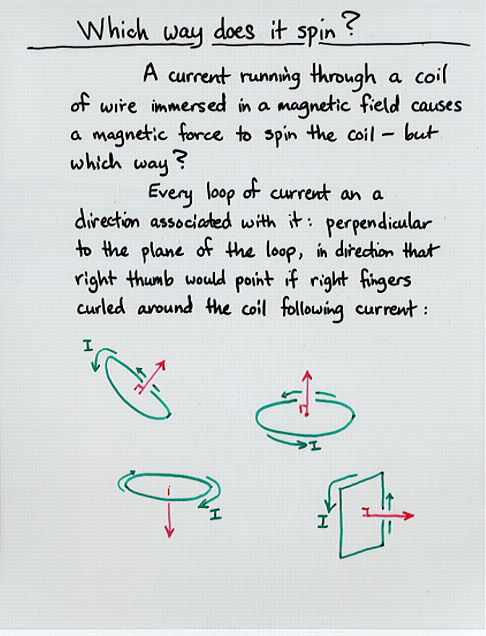Viewgraph 3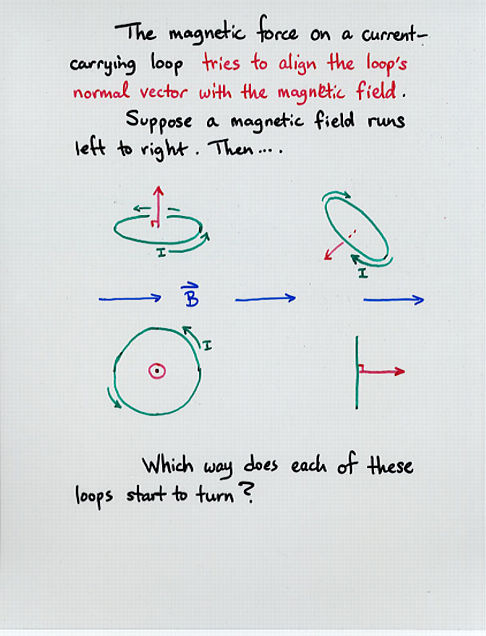Viewgraph 4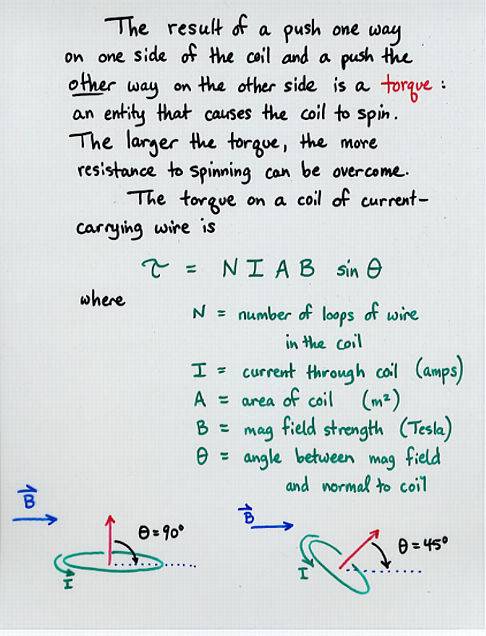Viewgraph 5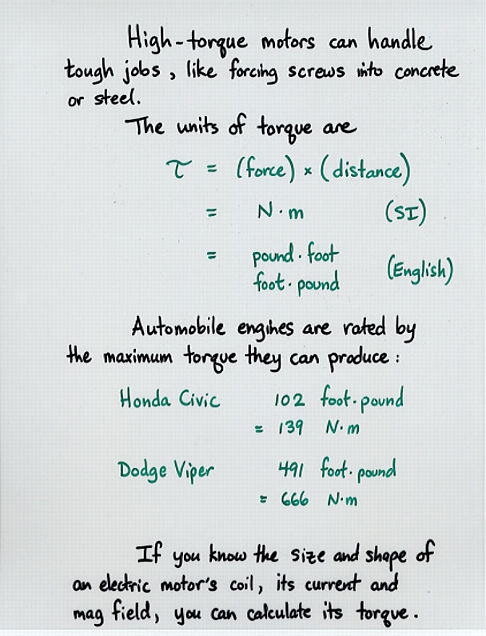Viewgraph 6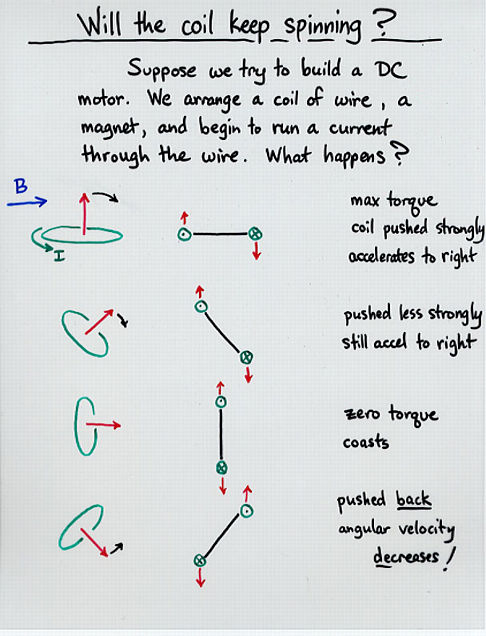Viewgraph 7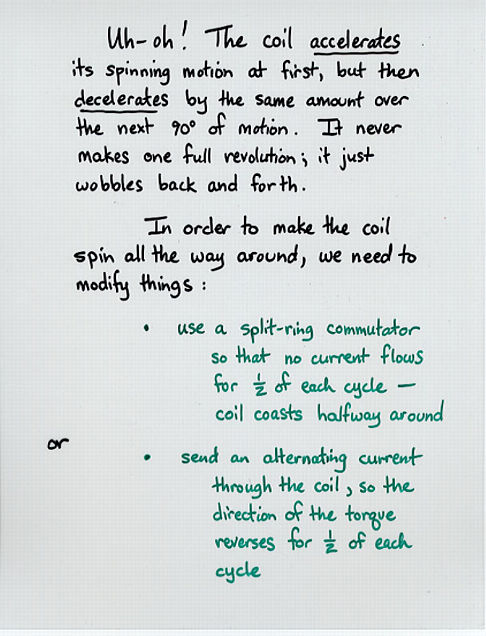Viewgraph 8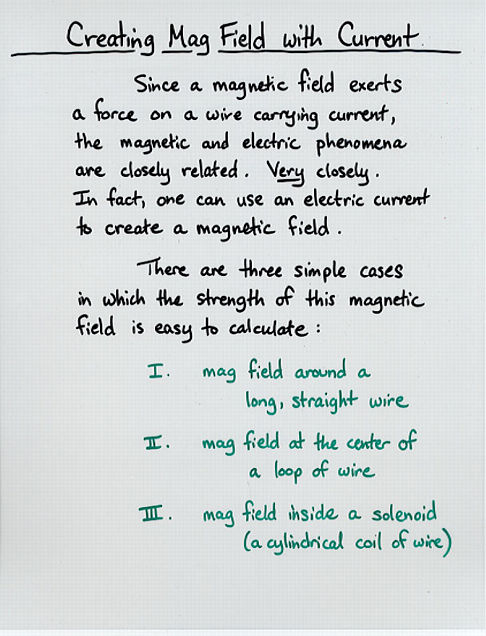Viewgraph 9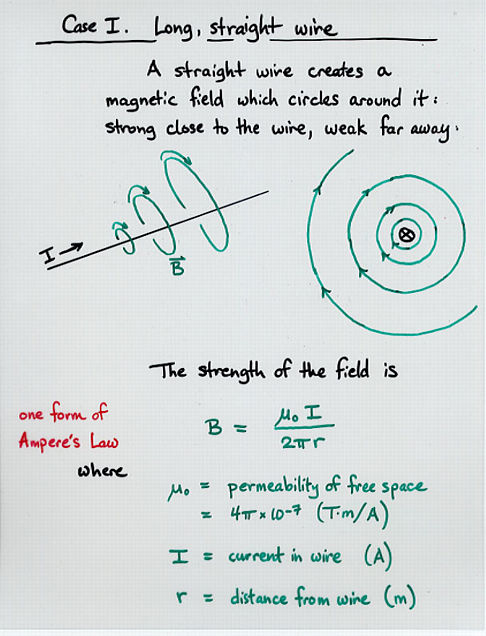Viewgraph 10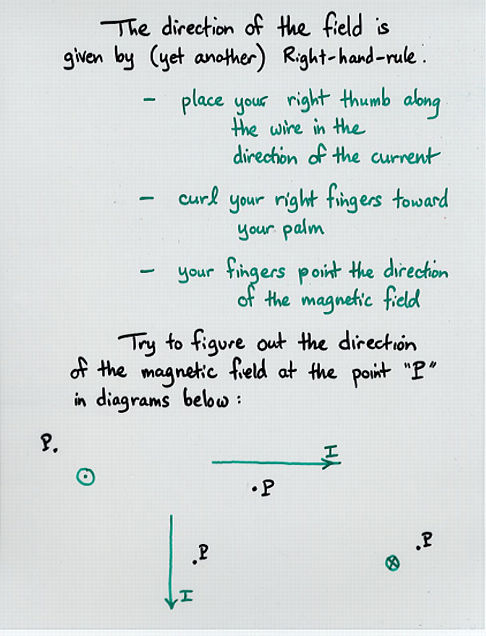Viewgraph 11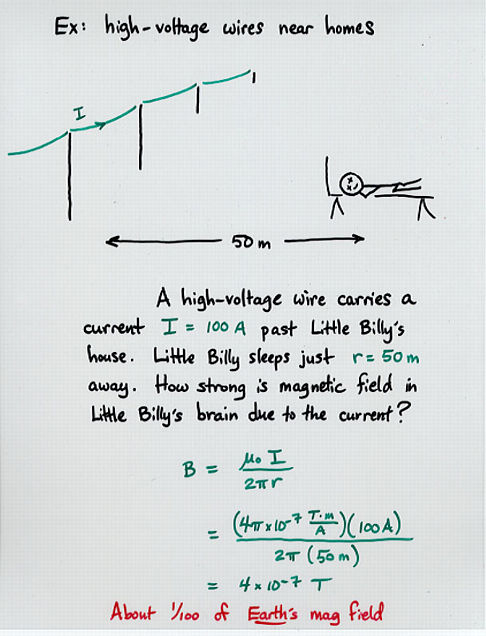Viewgraph 12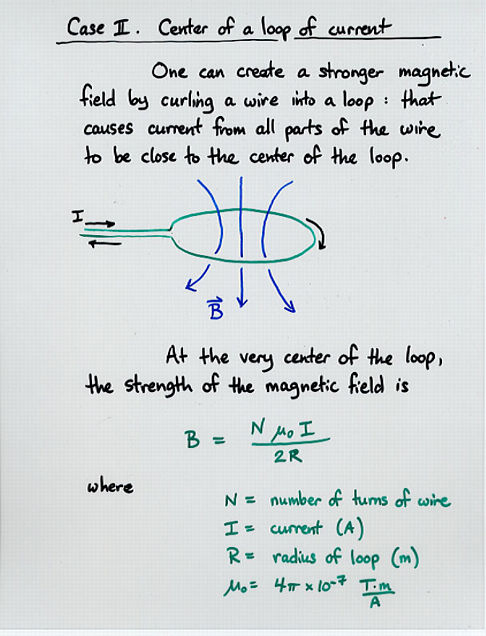Viewgraph 13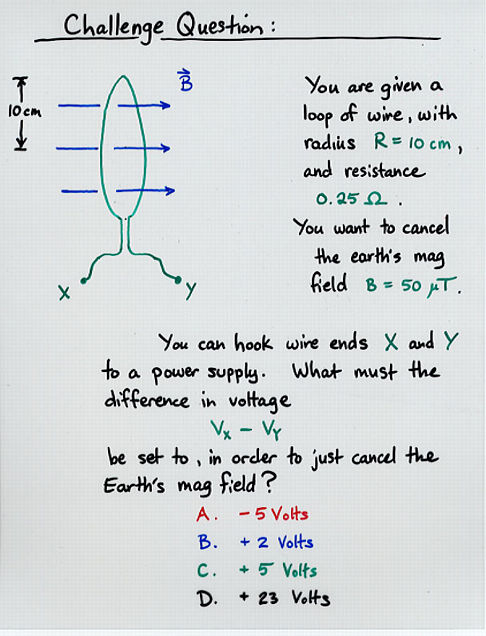Viewgraph 14Copyright © Michael Richmond. This work is licensed under a Creative Commons License.